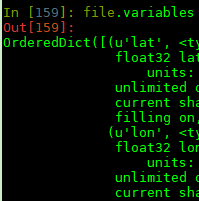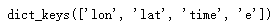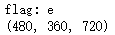• ## python读取.nc数据

万次阅读 2018-12-29 10:37:16
要用python读取.nc数据，首先要安装netCDF4包。运行 pip install netCDF4 即可。首先通过 import netCDF4 as nc file = nc.Dataset(file_path) 打开文件，如果我们对.nc文件的内容一无所知，那么可以通过 ...
要用python读取.nc数据，首先要安装netCDF4包。运行
pip install netCDF4
即可。首先通过
import netCDF4 as nc
file = nc.Dataset(file_path)
打开文件，如果我们对.nc文件的内容一无所知，那么可以通过
file.variables
命令，查看文件内容，可以查看保存文件数据的key、dtype、shape等信息，如下图最后，通过
data = file[key][:]
即可获取数据。

展开全文• # 读取nc文件 dataset = Dataset('sst.month.mean.nc', mode='r', format='NETCDF4') # 查看信息 print(dataset) 可以看到该文件共四个变量：lat, lon, time, sst. 查看其信息： # 查看变量 print(dataset....
拿到了一份历年全球海温的数据，格式是.nc，于是来读取看看。
from netCDF4 import Dataset
import numpy as np
# 读取nc文件
dataset = Dataset('sst.month.mean.nc', mode='r', format='NETCDF4')
# 查看信息
print(dataset)可以看到该文件共四个变量：lat, lon, time, sst. 查看其信息：
# 查看变量
print(dataset.variables.keys())查看某个变量的信息：
# 查看某个变量的信息
print(dataset.variables['sst'])查看某个变量的属性：
# 查看某个变量的属性
print(dataset.variables['sst'].ncattrs())查看变量具体的值：
# 查看变量的值
print(dataset.variables['sst'][:])可以看出来sst是一个f(lon, lat, time)的变量。
展开全文• 目前很多数据nc格式存储，下面这篇文章主要给大家介绍了关于利用python如何处理nc数据的相关资料，文中通过示例代码介绍的非常详细，对大家的学习或者工作具有一定的参考学习价值。需要的朋友们下面来一起看看吧
• ## python读取nc数据

千次阅读 2021-03-06 17:14:13
python读取nc数据 读取nc数据主要是用到netCDF4库。 #最基础的读取 nc_obj = nc.Dataset(filename) #这里filename自己定义即可 这里已经将nc文件读取进来了，之后我们可以查看其中的各个维度以及数据。 #读取经度...
python读取nc数据
读取nc数据主要是用到netCDF4库。
#最基础的读取
nc_obj = nc.Dataset(filename)
#这里filename自己定义即可

这里已经将nc文件读取进来了，之后我们可以查看其中的各个维度以及数据。
#读取经度纬度值
lat=(nc_obj.variables['lat'][:])
lon=(nc_obj.variables['lon'][:])

这里variables方法即是读取变量的功能，一般nc文件中经度和纬度会命名为‘lon’和‘lat’，不确定的话可以阅读一下相关产品的说明书进行查询。 或者可以直接调用variables.keys方法：
print(nc_obj.variables.keys())

输出结果：这里的变脸分别是经度、纬度、时间和蒸散发量。 那么我们想读取蒸散发数值的话，就是和前面类似的操作：
Data=(nc_obj.variables[str(flag)][:])

看一下读出来的结果的形状：
print(Data.shape)这个产品的记录是以月份为单位，一共是40年的。第二个是纬度，第三位是经度，都是以0.5°为单位。 到这里，数据已经存入数组Data中了，我们可以对它进行后续的操作。
补充一个题外话，如果想要灵活一点，让我们的程序可以每次读取我们选定的文件，而不需要我们每次更改路径，则可以使用win32ui库。
import win32ui
# 0代表另存为对话框，1代表打开文件对话框
dlg = win32ui.CreateFileDialog(1)

# 默认目录
dlg.SetOFNInitialDir('D:\Recently\Water_recycle\Data')

# 显示对话框
dlg.DoModal()

# 获取用户选择的文件全路径
filename = dlg.GetPathName()

nc_obj = nc.Dataset(filename)


这部分文件读取的内容一开始是在CSDN上找到的，但是后来找不到了，所幸我的程序里还留着，所以就搬运了，如果有朋友找到了之前那个大佬的帖子，麻烦指一下路，我补充一下应用，非常感谢！！
展开全文编程语言
• 有错误欢迎提出指正！ 两个月前大气环境模式上机作业，部分库的下载有点点麻烦（好像...import netCDF4 as nc import matplotlib.pyplot as plt import cartopy.crs as ccrs import pandas as pd import cartopy.featur
有错误欢迎提出指正！ 两个月前大气环境模式上机作业，部分库的下载有点点麻烦（好像是cartopy），不能直接用anaconda下载。该代码里面有部分内容是画累积降水的，没有删除，但是对运行这个没有影响o(￣▽￣)ブ

感谢导师袁老、陈x同学、以及小男友对俺的技术指导

import netCDF4 as nc
import matplotlib.pyplot as plt
import cartopy.crs as ccrs
import pandas as pd
import cartopy.feature as cfeature
from cartopy.mpl.gridliner import LONGITUDE_FORMATTER, LATITUDE_FORMATTER
import matplotlib.ticker as mticker
import numpy as np
from matplotlib.patches import Rectangle
import matplotlib

plt.rcParams['font.family'] = ['sans-serif']
plt.rcParams['font.sans-serif'] = ['SimHei']
#读取文件
file = r'H:\wrfout.nc'
dataset =nc.Dataset(file,'r') #读取nc文件
Rain=dataset.variables['RAINNC'][:,::-1] #需要先找出nc文件里面的变量名再赋值
T2=dataset.variables['T2'][:,::-1]
lon=dataset.variables['XLONG'][:]
lat=dataset.variables['XLAT'][:,::-1]
#print(dataset.variables['T2'])
lon_need=np.array(lon[115:130,117:132])#这个区域是我根据降水分布图找到的降水量特大区，然后自己指定的该区域
lat_need=np.array(lat[115:130,117:132])
fig=plt.figure(figsize=(12,10),dpi=550)
#绘制3小时气温
fig.suptitle('整个模拟区域逐3小时气温',fontsize=8)
fig.suptitle('指定区域逐3小时气温(UTC)',fontsize=8)

levels=np.arange(20,30,1)
for i in range(1,10):

a=12+3*(i-1)
if a>24:
ax.set_title('22日{}时'.format(a-24),fontsize=3,loc='left',y=0.8)
else:
ax.set_title('21日{}时'.format(a),fontsize=3,loc='left',y=0.8)

#地理信息
shp_path_1=r'E:\WRF\区划\省.shp'
enshicity_1 = cfeature.ShapelyFeature(reader_1.geometries(), crs=ccrs.PlateCarree(), edgecolor='k', facecolor='none')
##经纬度范围
#extent=[105,120,20,30]
extent=[108,111,24,26]
ax.set_extent(extent)

#绘制经纬度
gl = ax.gridlines(crs=ccrs.PlateCarree(), draw_labels=True, linewidth=0.2, color='k', alpha=0.5, linestyle='--')
gl.top_labels = False ##关闭上侧坐标显示
gl.right_labels = False ##关闭右侧坐标显示
if i !=1 and i!=6 :
gl.left_labels=False

gl.xformatter = LONGITUDE_FORMATTER ##坐标刻度转换为经纬度样式
gl.yformatter = LATITUDE_FORMATTER
gl.xlocator = mticker.FixedLocator(np.arange(extent, extent, 1))
gl.ylocator = mticker.FixedLocator(np.arange(extent, extent, 1))
gl.xlabel_style={'size':3}
gl.ylabel_style={'size':3}
T = T2-273.15
b = ax.contourf(lon,lat,T[i*3-3],cmap='Spectral_r',levels=levels,extend='both')
x = ax.plot(lon_need, lat_need, color='b', linewidth='0.5', linestyle='--')
y = ax.plot(lon_need, lat_need, color='b', linewidth='0.5', linestyle='--')
m = ax.plot(lon_need[:, 0], lat_need[:, 0], color='b', linewidth='0.5', linestyle='--')
n = ax.plot(lon_need[:, 14], lat_need[:, 0], color='b', linewidth='0.5', linestyle='--')

cb=plt.colorbar(b, cax=position,extend='both',orientation='horizontal')
font = {'family' : 'serif',
'color'  : 'darkred',
'weight' : 'normal',
'size'   : 6,
}
cb.ax.tick_params(labelsize=5)
plt.subplots_adjust(left=0.110, bottom=0.205, right=0.900, top=0.835, wspace=0.165, hspace=0)

plt.show()

欢迎各位同行的指正与讨论~
展开全文• .nc（network Common Data Format）文件是气象上常用的数据格式，python读取.nc使用较多的库为netCDF4这个库，下面将介绍这个库的具体方法。 安装很简单: pip install netCDF4 .nc文件介绍参考链接：...
• 库xarray可以帮我们读取出nc文件的内容，并生成dataset，我们通过取dataarray，通过计算来达到各种目的。 那么，要如何通过xarray读出nc文件内容并简简单单画个图呢？以下是我的学习心得： 第一步我使用open_...
• dataset = nc.Dataset('xxx.nc') # 读取数据 print(dataset.variables.keys()) # 输出所有变量 lon = dataset.variables['longitude'][:].data # 读取经度 lat = dataset.variables['latitude'][:].data # 读取维度 ...
• nc文件读取 python语言编写的 读取nc文件
•   NetCDF(network Common Data Form)网络...  这里采用python的一个专门用来处理.nc文件的库–netCDF4 该库的安装直接: pip install netCDF4 这个库玩起来稍微比Pandas复杂一些。 下面以全球降水量数据为例进行可视化
• 今天小编就为大家分享一篇python读取与处理netcdf数据方式，具有很好的参考价值，希望对大家有所帮助。一起跟随小编过来看看吧
• ## python读取nc文件

万次阅读 多人点赞 2018-10-26 10:15:43
我这两天折腾用python读取nc文件，查阅很多资料，左拼右凑的终于读出来了。 1、安装Anaconda 1）Anaconda的安装这里有详细的讲解。搜索“Anconda”，进入官网下载。我的电脑上安装的是python3.7，因此下载的...
• Temperature=ncin.variables['Temperature'] Temperature #提取单个变量，详细显示变量所有信息 print(Temperature[:]) #显示数组所有元素python
• 然后安装读nc文件所需的模块netCDF4：conda install netCDF4 这样就完成了HDF4模块的安装。 # -*- coding: utf-8 -*- """ Spyder Editor This is a temporary script file. """ import netCDF4 from netCDF4 ...
• 从nasa MODIS官方网站通过FTP获取的日数据，用于分析海洋叶绿素浓度，其数据格式为 .nc文件， This algorithm returns the near-surface concentration of chlorophyll-a (chlor_a) in mg m-3, calculated using an...
• # 读取nc数据 dataset = Dataset(source_file) print(dataset.variables.keys()) # 获取相应数组集合--纬度经度温度深度 # Dimension time = nc_obj.variables['time'][:] lev = nc_obj.variables['depth']...
• 使用MATLAB读取nc文件格式代码，提取数据，完成作图等。
• nc和hdf是一家人，5行Python代码一锅端netCDF4
• 若不想阅览下方nc数据描述部分，可跳转至：查看变量信息 最后附上了完整代码。 查看变量信息 第十八行开始逐个查看每个字段的描述，由于过多此处没有截取完全 最终确定需要字段为solar_mon 完整代码 ...编程语言
• ## python netcdf4读取nc格式的气象数据

万次阅读 多人点赞 2019-12-04 17:03:06
二、nc格式数据读取 #!usr/bin/env python # -*- coding: utf-8 -*- """ @Author : zhaoguanhua @Email : zhaogh@hdsxtech.com @Time : 2019/12/4 16:45 @File : weather.py @Software: PyCharm """ im......

# python读取.nc数据python 订阅